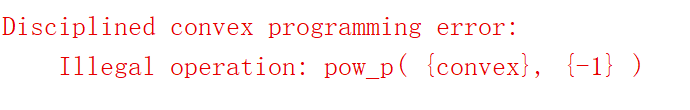# How can I minimize the function in the form of 1/(x^3-1), with x>1?

The objective function is surely convex as its second derivative is (6x*(2x^3 + 1))/(x^3 - 1)^3 which is positive if x>1. However, the cvx failed to optimize the problem. How can I deal with the function? A test code is shoed as follows
cvx_begin
variable x;
minimize inv_pos(x^3-1);
subject to
x>=1;
y<=10;
x+y<=3;
cvx_end

And the error message is

You could

maximize x

Thank you for replying. I’m sorry that my example may be not appropriate. What about this objective function? It is still convex. But cvx failed to solve it. How can I optimize it?
cvx_begin
variable x;
variable y;
minimize inv_pos(pow_p(x,3)-1)+inv_pos(pow_p(y,3)-1);
subject to
x>=1;
y>=1;
x+y<=3;
cvx_end
Here is the error messageThanks a lot again.

You can do
minimize(pow_p(x,-3)+pow_p(y,-3))

As for 1/(x^3-1) for x > 1, it appears that none of the forum readers know how to formulate that in CVX, despite its convexity. Therefore, it is a candidate for @Erling’s challenge. https://twitter.com/J_P_Vielma/status/1014513695605608448

Edit: I stand corrected on the preceding paragraph in light of @Michal_Adamaszek’s post below.

t\geq \frac{1}{xyz-1}, \ xyz>1

of which the present question is a special case.